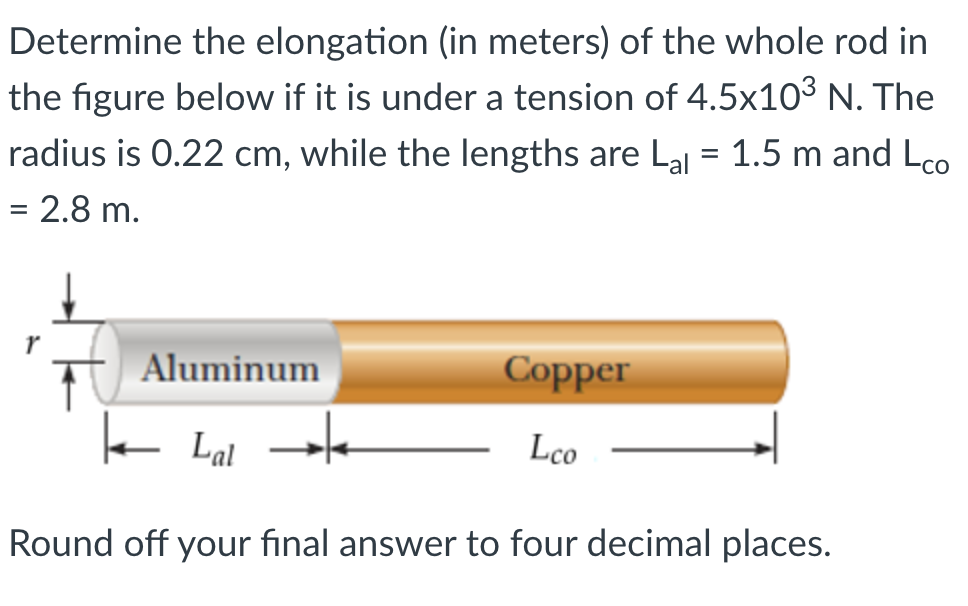### Create an Account

Home / Questions / Determine the elongation (in meters) of the whole rod in the figure below if it is under a...

# Determine the elongation (in meters) of the whole rod in the figure below if it is under a tension of 4.5x103 N. The radius is 0.22 cm, while the lengths are Lal = 1.5 m and Lco = 2.8 m. Copper

Determine the elongation (in meters) of the whole rod in the figure below if it is under a tension of 4.5x103 N. The radius is 0.22 cm, while the lengths are Lal = 1.5 m and Lco = 2.8 m. Copper Aluminum I la Lco Round off your final answer to four decimal places.May 27 2021 View more View LessSubscribe To Get Solution